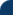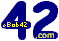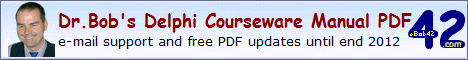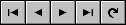Delphi Clinic C++Builder Gate Training & Consultancy Delphi Notes Weblog Dr.Bob's WebshopDr.Bob Examines... #105 See Also: Dr.Bob's Delphi Papers and ColumnsDelphi 2009 Anonymous Methods
In this article, I'll examine Delphi 2009 Anonymous Methods. An anonymous method is a method without a name; hence it's anonymous. In fact, you can see it as a chunk of code that has been copied and can be pasted anywhere else. In general, an anonymous method is great for situations where a normal for-in-loop doesn't work. But in this section, I'll do my best to give some examples of places where anonymous methods can be useful.
As first example, consider the following definition of a procedure reference, which can be called, and assigned directly, without specifying a name. In fact, the assignment is just a block of code, nothing more:

```  type
TProc = reference to procedure(x: Integer);

procedure call(const proc: TProc);
begin
proc(42);
end;

var
proc: TProc;
begin
proc := procedure(a: Integer)
begin
Button1.Caption := IntToStr(a)
end;
call(proc)
end;
```
This is just a first example, and it may not feel that natural, so let's continue with some more places where we can use anonymous method.

Anonymous Exit
Using anonymous methods in combination with Exit, you can write code that has no more need for else statements (or local routines for that matter). I've marked the anonymous method again, to make it a bit clearer where the actual code surrounding the anonymous method is, and what the anonymous method itself consists of:

```  function Power(const Value: Extended; Exponent: Integer): Extended; overload;
type
TMultiply = reference to function(const v: Extended; e: Integer): Extended;
var
Times: TMultiply;
begin
if Value = 0 then Exit(0);
if Exponent = 0 then Exit(1);
Times := function(v: Extended; e: Integer): Extended
begin
Result := v;
while e > 0 do Result := Result * v
end;
if Exponent < 0 then
Exit(1 / Times(Value,Exponent+1));
Result := Times(Value,Exponent-1)
end;
```
This combination of anonymous methods and Exit is actually leading to code which is very hard to read (and maintain), so although it's possible, I would certainly not recommend (ab)using anonymous methods in this way.

Generics.Defaults
The unit Generics.Defaults is included with Delphi 2009 and contains a number of pre-defined interface and class definitions. Note that if you want to examine the details of unit Generics.Defaults when placed in a uses clause, you need to press down the control key and click on the Defaults part, not on the Generics part. The first definition is for IComparer<T>, an interface that defines a Compare function, taking Left and Right arguments of type T, returning an integer value (< 0 if left smaller than right, > 0 is left is bigger than right).

```  type
IComparer<T> = interface
function Compare(const Left, Right: T): Integer;
end;
```
We can use this interface when we need to compare generics, as I'll show in a minute. Implementing this interface, means writing a Compare function. However, using anonymous methods, we can pass a reference to a function with the correct signature. That's why we also have a definition for TComparison<T> being a reference to a function with the Compare signature:
```   TComparison<T> = reference to function(const Left, Right: T): Integer;
```
To complete the story, Generics.Defaults also contains an abstract TComparer<T> class, implementing the IComparer<T> interface. The TComparer<T> class also includes two class functions that return the IComparer<T> interface:
```  TComparer<T> = class(TInterfacedObject, IComparer<T>)
public
class function Default: IComparer<T>;
class function Construct(const Comparison: TComparison<T>): IComparer<T>;
function Compare(const Left, Right: T): Integer; virtual; abstract;
end;
```
The Construct class function uses the anonymous method way to allow us to pass a TComparison<T> in order to return an IComparer<T> that we can use to compare two T values.

IEqualityComparer<T> is an interface that defines an Equals function (taking a Left and Right argument of type T again), as well as a GetHashCode function. This interface can be used to compare two generic values in order to check if they are equal. To support this interface, two function references were defined:

```  TEqualityComparison<T> = reference to function(const Left, Right: T): Boolean;

THasher<T> = reference to function(const Value: T): Integer;
```
Obviously, we can use anonymous methods to define "in-place" code where these references are used.
To complete the equality story, there is an abstract TEqualityComparer<T> class, implementing the IEqualityComparer<T> interface. The TEqualityComparer<T> class is defined as follows:
```  TEqualityComparer<T> = class(TInterfacedObject, IEqualityComparer<T>)
public
class function Default: IEqualityComparer<T>; static;

class function Construct(const EqualityComparison: TEqualityComparison<T>;
const Hasher: THasher<T>): IEqualityComparer<T>;

function Equals(const Left, Right: T): Boolean;
reintroduce; overload; virtual; abstract;
function GetHashCode(const Value: T): Integer;
reintroduce; overload; virtual; abstract;
end;
```
The Generics.Defaults unit contains more definitions, including a non-reference counted IInterface implementation in TSingletonImplementation, featuring implementations of QueryInterface, _AddRef, and _Release.

Also included are a TDelegatedComparer<T> and TDelegatedEqualityComparer<T> which are required, since the TComparer<T> and TEqualityComparer<T> are abstract base classes. Finally, we can also find the classes TCustomComparer<T> and TStringComparer (specific for Strings).

Delphi 2009 Development Essentials
This article is an excerpt from my Delphi 2009 Development Essentials courseware manual which has been sent in PDF format to all my clients, and which is sold at Lulu.com.

This webpage © 2008-2010 by Bob Swart (aka Dr.Bob - www.drbob42.com).All Rights Reserved.